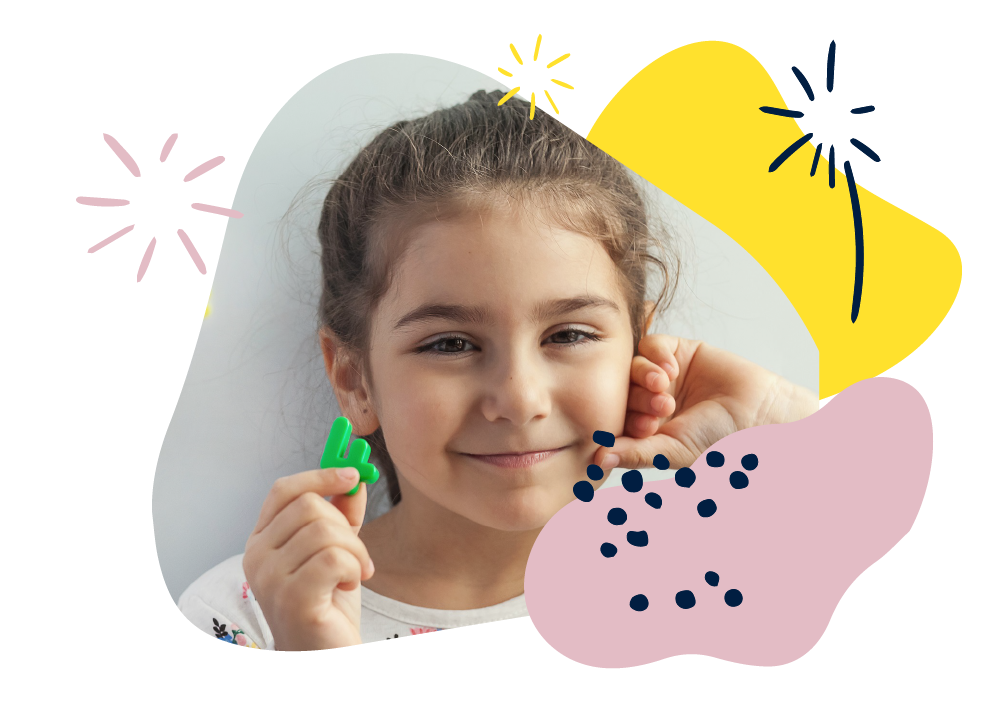We use cookies to enhance your experience on our website. By continuing to use our website, you are agreeing to our use of cookies. You can change your cookie settings at any time. Find out more# Maths: Age 8–9 (Year 4)

In Year 4, your child will develop their mental and written calculation skills using larger positive numbers and fractions. They will meet negative and decimal numbers, as well as some Roman numerals. They will convert between units of measurement, find perimeters and areas, and learn more about angles and symmetry. They will plot shapes on coordinate grids and start to use time line graphs.

They’ll count in steps of 6, 7, 9, 25 and 1000, add and subtract four-digit numbers and multiply three-digit by one-digit numbers. They will know all the times tables up to 12 x 12 and be able to divide by 10 and 100 to give decimal answers. They’ll round numbers to the nearest 10, 100 and 1000, or whole number for decimals.

Don’t worry if some methods that your child learns are new to you! There are still simple things you can do at home to support their development.

## How to help at home

You don’t need to be an expert to support your child with maths or help them develop a good sense of number! Here are three simple, but effective learning ideas that you can try with your child using everyday items at home.

### 1. Find the negative!

Look for negative numbers to discuss with your child. For example, thermometers, weather reports, storage temperatures on frozen food packets, elevation of places below sea level, goal differences in sports league tables, below-ground floors in lifts, losing points in video games.

### 2. Play times tables games

Make two sets of number cards 1–12. Shuffle and place them face down in two piles. Take a number from each pile and multiply them together. Score a point for each correct answer. The first to 10 points wins.

### 3. Round at the shops

Practise rounding decimals with one decimal place to the nearest whole number when shopping. Challenge your child to round prices to the nearest pound. For example, an item costing £4·70 would be £5 to the nearest pound.

You’ll find more activity ideas in our Kids’ activities section

## What your child will learn at school

#### Number & place value in Year 4 (age 8–9)

In Year 4, children will be expected to order and compare numbers beyond 1000 using place value in 4-digit numbers (for example, 1423 has 1 thousand, 4 hundreds, 2 tens and 3 ones). This includes:

• counting in steps of 6, 7, 9, 25 and 1000
• counting backwards through zero to include negative numbers
• rounding any number to the nearest 10, 100 or 1000.

#### Addition & subtraction in Year 4 (age 8–9)

In Year 4, children will be expected to be able to solve addition and subtraction problems involving numbers up to four digits. This includes:

• choosing from a variety of methods, including mental calculations, using objects, diagrams or drawings such as number lines, models such as the area/grid method or written column addition and column subtraction
• estimating answers before calculating accurately and checking answers by understanding that addition and subtraction are inverse operations
• solving two-step word problems which require them to solve two different calculations before coming to the answer.

#### Multiplication & division in Year 4 (age 8–9)

In Year 4, children will be expected to be able to use formal written methods of short multiplication and short division confidently. This includes:

• knowing and using multiplication and division facts for all times tables up to 12 × 12
• multiplying three-digit by one-digit numbers
• multiplying three numbers together.

#### Fractions & decimals in Year 4 (age 8–9)

In Year 4, children will be expected to explore the link between fractions and multiplication/division and begin to learn about decimals. This includes:

• solve simple measure and money problems involving fractions and decimals
• recognising and writing tenths and hundredths as decimals
• rounding decimal numbers with one decimal place to the nearest whole number.

#### Geometry in Year 4 (age 8–9)

In Year 4, children will be expected to extend their knowledge of properties of shapes and be able to describe the position of shapes on a coordinate grid. This includes:

• comparing shapes such as quadrilaterals and triangles based on their properties and sizes
• drawing mirror images of shapes in lines of symmetry
• plotting points and describing how shapes move up/down/left/right on a coordinate grid.

#### Measurement in Year 4 (age 8–9)

In Year 4, children will be expected to be able convert between some units of measurement, such as kilometres to metres and calculate measurements in relation to shapes. This includes:

• working out the perimeter and area of rectilinear shapes
• solving problems involving converting units of time
• adding, subtracting, multiplying and dividing measurements including money.